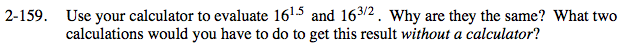### Home > PC > Chapter 2 > Lesson 2.3.7 > Problem2-159

2-159.

Use your calculator to evaluate 161.5 and 163/2. Why are they the same? What two calculations would you have to do to get this result without a calculator? Homework Help ✎$16^{3/2}=16^{1.5}=64$

They are the same because

$1.5=\frac{3}{2}$